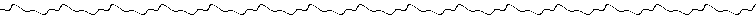LESSON1:

To make an ASM program you will need the foloowingfiles:
• ASM86v?? (found on ticalc.org)
• TASM (also found on ticalc.org)
• TASM80.TAB (notice a patern)
After you have all the programming utilities you need,you are almost ready to start your first program. This is what every ASM program will look like:

#include "asm86.h"        ;I use Asm86 to compile, so I include this
#include "ti86asm.inc"     ;this is TI's includefile

.org _asm_exec_ram         ;starting point for all asm progs, \$D748
; all the ASM goes in here
.end

The most important thing you need to understandis what a register is, and how to use it.  A register is a 16bit (2byte) memory location, found on the CPU itself.  On the Z80 you havethe following important Registers:
• AF
• BC
• DE
• HL
• IX
• IY
• (there are others, but we won't use them in my examples,I don't even unstand them all)
These registerscan be broken up into TWO 8bit (1 byte) registers each. (ie A,F,B,C,D,E,L,..) Of these 8 bit registers, A is the most important.  Register A iswhat is called the Accumulator, some calls will only work on A (sub,or,xor,and,...). The A register is grouped with the F register.. Why? well F stands forFlags, and it contains the follwing flags (sign,zero,half carry,parity,add/subject,carry)Theseare the instructions that will be covered in this lesson:
LD
INC/DECLD
Thefirst thing you will want to do is to load a value into a register. This is done with the LD instruction.  Here are some examples:
ld a,0    ; loads the value0 into register a
ld b,2    ; loads the value 2 intoregister b
ld de,257 ; loads the value 257 into register de
ld d,\$0A  ; NOTE \$8A represents a HEX number,
; %00100100 represents a BIN number,
; 52 just a decimal number.
; this loads \$0A into d, \$0A is the same as 10
ld a,d    ; loads the current valueof d into a (in this case 10)

NOTE:An 8-bit regiser can only hold the values from 0-255 (%00000000-%11111111),but a 16 bit register can hold the values 0-65535.
The register HL, is primarly used for ADDRESSING,this means it usually points to another memory location.  The videoMemory is located at \$FC00, so to have hl "point" to the video memory youuse the command:
ld hl,\$FC00 ;loads the value \$FC00 into registerhl
Now, to copy a value into the memory locationthat HL is pointing to, we do somthing called inderect addressing. This is accomplished by placing parens around the register name.
ld a,%10101010    ;loads thevalue %10101010 into reg. a
ld (hl),a        ;loads the value %10101010 into the
;memory location that hl "points" to
;the value of HL is \$fc00 therefore
;the value %10101010 is loaded into
;memory location \$fc00, which happens
;to be the video memory :)
;IT DOES NOT CHANGE THE VALUE OF HL!!
ld a,(hl)        ;similiarly this loads the value at
;mem location \$fc00 into the reg. a

back to list of instructionsThe next thing to learn, is how to add and subtractfrom a register.  To do this we use the indtructions ADD and SUB.
• add hl,ss  (where ss = bc,de,hl, or sp)
• add ix,pp (where pp = bc,de,ix, or sp)
• add iy,rr  (where rr = bc,de,iy, or sp)
These are the only ways that ADD can be used.  ex:
ld a,8      ;a=8
ld hl,\$FC00 ;hl = \$FC00
ld bc,\$00BB ;bc = \$00BB
add hl,FCBB ;hl=hl+bc  hl = \$fcbb

In order to add anything to the other registers, you mustdo it inderectly:
ld b,8      ;b=8
ld a,b      ;a=b
ld b,a      ;b='b+5'

;or

ld bc,46    ;bc=46
ld h,b      ;you can't do'ld hl,bc'
ld l,c      ;
ld bc,52    ;
ld b,h      ;.
ld c,l      ;bc =bc+52

• sub ##  ; a=a-##
That's it!  you can only SUB from a (the accumulator),therefore all other subratcions must be made inderectly.  Here aresome examples:
ld a,16    ;a=16
sub 5      ;a=a-5, a=11

ld b,65    ;b=65
ld a,b     ;a=65
sub 6      ;a=65-6, a=59
ld b,a     ;b=59

ADD and SUB let you add or subtract any number, however ifyou only want to add or subract the value 1 then you can use INC/DEC

back to list of instructionsINC/DEC
• inc r   ;(where r=a,b,c,d,e,h,orl)
• inc ss  ;(where ss=bc,de,hl, or sp)
• dec r
• dec ss
These are the ony cases we will use.  Here are someexamples:
ld a,5    ;a=5
inc a     ;a=a+1, a=6
ld b,a    ;b=a, b=6
dec b     ;b=b-1, b=5

ld bc,568 ;bc=568
inc bc    ;bc=bc+1, bc=569
inc bc    ;bc=bc+1, bc=570

back to list of instructionsINDEX    LESSON2
This is the end of Lesson1, I do not gaurantee any correctnessof any statements made above.